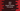# Find the largest number from an array in Java## Java program to find the largest number that can be formed from an array:

In this post, we will lean how to find the largest number that can be formed from an array in Java. The program will take the array numbers as inputs from the user and it will print the largest number that can be developed from that array.

### Algorithm:

Let’s consider the below array:

``[1, 8, 5, 7, 9]``

The largest number that we can formed using the above array is:

``98751``

We can either keep iterating through the array, get the largest value and add it to build the final number. But the easiest way to do this is by sorting the array.

For example, if I sort the above array, it will become:

``[1, 5, 7, 8, 9]``

We can then iterate through the numbers of the array from last to start and combine them to create the final value.

To create the final number, initialize one variable finalValue as 0. Iterate through the items of the array from end to start and for each value found, multiply finalValue with 10 and add that value as the new value for finalValue.

If I am iterating through the numbers of the sorted array [1, 5, 7, 8, 9] and calculate the finalValue, it will look like:

``````For 9, finalValue = 0 * 10 + 9 = 9
For 8, finalValue = 9 * 10 + 8 = 98
For 7, finalValue = 98 * 10 + 7 = 987
For 5, finalValue = 987 * 10 + 5 = 9875
For 1, finalValue = 9875 * 10 + 1 = 98751``````

So, finally finalValue will hold the largest number that can be built from the given array.

That’s it. Let’s write it in code.

### Java program:

Below is the complete java program:

``````package com.company;

import java.util.Arrays;
import java.util.Scanner;

public class Example {
private static int getMaxNumber(int[] arr) {
Arrays.sort(arr);

int finalValue = 0;

for (int i = arr.length - 1; i >= 0; i--) {
finalValue = finalValue * 10 + arr[i];
}

return finalValue;
}

public static void main(String[] args) {
int size;
Scanner sc = new Scanner(System.in);

System.out.println("Enter the size of the array :");
size = sc.nextInt();

int[] givenArr = new int[size];

System.out.println("Enter the numbers for the array:");
for (int i = 0; i < size; i++) {
givenArr[i] = sc.nextInt();
}

System.out.println(getMaxNumber(givenArr));
}
}``````

Here,

• getMaxNumber is the main method used to get the maximum value that we can build from the provided array arr.
• In main, we are taking the size of the array and storing it in size. Then, we are creating one array givenArray of size size and taking the array numbers as input from the user one by one. These numbers are inserted in the array givenArray.
• Finally, it is calling getMaxNumber with the array passed and printing its return value, i.e. the maximum number that can be build from the array.
• In getMaxNumber, it is first sorting the array and building the final number finalValue as we have seen above.

It will print output as like below:

``````Enter the size of the array :
5
Enter the numbers for the array:
9 4 8 2 1
98421``````